Courses

# Worksheet: Pair of Linear Equations in Two Variables Class 10 Notes | EduRev

## Class 10 : Worksheet: Pair of Linear Equations in Two Variables Class 10 Notes | EduRev

The document Worksheet: Pair of Linear Equations in Two Variables Class 10 Notes | EduRev is a part of the Class 10 Course Mathematics (Maths) Class 10.
All you need of Class 10 at this link: Class 10

1. Solve the system of equations, using crossmultiplication method:
2x + 5y = 1

x + 3y = 3

2. Solve for x and y: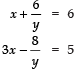3. Solve for x and y: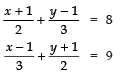4. Solve the following system of equation graphically:
2x + 3y = 8
x + 4y = 9

5. Two numbers are in the ratio 5 : 6. If 8 is subtracted from each of the numbers, the ratio becomes 4 : 5. Find the numbers.

6. The cost of 4 pens and 4 pencil boxes is ₹ 100. Three times the cost of a pen is ₹ 15 more than the cost of a pencil box. From the pair of linear equations for the above situation, find the cost of a pen and a pencil box.
Hint: Let x is cost of a pen and y is the cost of a pencil box, then 4x + 4y = 100
3x  = y + 15
Solving we find:
Cost of a pen = ₹. 10 and cost of pencil box = ₹. 15

7. Solve the following system of equations graphically:
(i) x − 2y = 4
x − y = 3

(ii) x + 2y = 3
4x + 3y = 2

8. Solve the following system of equations graphically:
2x + 3y = 2
x − 2y = 8

9. Solve for x and y: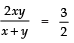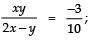x + y ≠ 0 and 2x – y ≠ 0

10. Solve for x and y: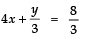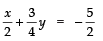11. Solve the following system of linear equations graphically:
2x + 3y =12
2y − 1= x

12. Solve the following systems of equations graphically:
2x − 3y = 1
4x − 3y + 1 = 0

13. Solve the following system of linear equations graphically:
3x − 4y − 12 = 0
x + 3y − 4 = 0

14. Solve for x and y:
47x + 31y = 63
31x + 47y = 15

15. Solve for x and y: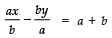ax − by =2ab

16. Solve for x and y:
4x − y − 8= 0
2x − 3y + 6 = 0

17. Draw the graph of the equations:
4x − 5y + 16 = 0
2x + y − 6= 0

18. Draw the graphs of the equations:
3x − y + 9 = 0
3x + 4y − 6= 0

19. Draw the graphs of the following equations:
3x − 4y + 6 = 0
3x + y − 9 = 0
Also determine the co-ordinates of the vertices of the triangle formed by three lines and x-axis.

20. Draw the graphs of the equations:
3x + 2y + 6 = 0
3x + 8y − 12 = 0
Also determine the co-ordinates of the vertices of the triangle formed by these lines and the x-axis.

21. Draw the graphs of the equations:
2x − y + 6 = 0
4x + 5y − 16 = 0
Also, determine the co-ordinates of the vertices of the triangle formed by these lines and the x-axis.

22. Solve the following system of linear equations graphically:
3x − 2y − 1= 0
2x − 3y + 6 = 0
Shade the region bounded by the lines and x-axis.

23. Solve the following system of linear equations graphically:
4x – y = 4
2x + 2y = 14
Shade the region hounded by the lines and y-axis.

24. Solve the following system of linear equations graphically:
3x + 2y – 4 = 0
2x – 3y – 7 = 0
Shade the region bounded by the lines and x-axis.

25. Solve the following equations graphically for x and y:

3x + 2y = 12
5x − 2y = 4
Find the co-ordinates of the points where the lines meet the y-axis.

26. Solve the following equations graphically:
3x − 4y = 7
5x + 2y = 3
Shade the region between the lines and the y-axis.

27. Solve graphically the pair of equations
2x + 3y = 11
2x − 4y = −24
Also, find the value of co-ordinates of the triangle so formed

28. The sum of the numerator and denominator of a fraction is 8. If 3 is added to both the numerator and denominator the fraction becomes 3/4, find the fraction.

29. Find the values of x and y in the following rectangle:
Hint: Opposite sides of a rectangle are equal
∴ x + 3y = 13
and 3x + y = 7
Solve the above system of linear equations.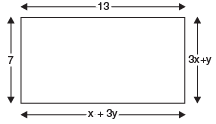30. The angles of a triangle are x, y and 40°.

The difference between the two angles x and y is 30°. Find x and y.
Hint: Since, the sum of three angles of a triangle is 180°
⇒ x + y + 40 = 180°
or
x + y = 180° – 40° = 140°
Also, difference between x and y is 30° ⇒ x – y = 30°
Now solving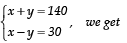x = 85°
and y = 55°

31. Graphically, solve the following pair of equations: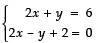Find the ratio of the areas of the two triangles formed by the lines representing these equations with the x-axis and lines with the y-axis.

32. In a bag containing white and red balls, half the number of white balls is equal to one third the number of red balls. Twice the total number of balls exceeds three times the number of red balls by 8. How many balls of each type does the bag contain?
Hint: Let the number of white balls be ‘x’ and the number of red balls be y.
⇒ Total no. of balls = (x + y)
∵ 1/2 [no. of white balls] = 1/3 [number of redballs]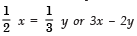Also, = 0     ...(1)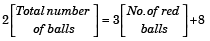⇒ 2(x + y) = 3y + 8 or 2x – y = 8   ...(2)

Multiplying (2) by 2 and subtracting the product from (1)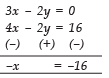From (1), 3(16) – 2y = 0 ⇒ y = 24 So, number of white balls is 16 and red balls is 24.

33. Solve the equations 5x – y = 5 and 3x – y = 3 graphically.
Hint: 5x – y = 5 3x – y = 3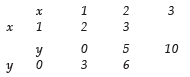(x,y)(1,0) (2,5) (3,10) (x,y) (1,0)(2,3) (3,6)
Joining the ordered pair, we get the graphical solution: x = 1; y = 0

ANSWERS
1. x = 3; y = –1

2. x = 3; y = 2

3. x = 7; y = 13

4. x = 1, y = 2

5. 40 and 48

6. ₹ 10 and ₹ 15

7.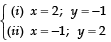8. x = 4; y = –2
9. x = 1/2, y = -3/2

10. x = 1; y = –4

11. x = 3; y = 2

12. x = –1; y = –1

13. x = 4; y = 0

14. x = 2; y = –1

15. x = b; y = –a

16. x = 3; y = 4

28. 3/5

29. x = 1; y = 4

30. x = 85°; y = 55°

31. x = 1, y = 4; 4 : 1

32. 16, 24

33. 1,0

Offer running on EduRev: Apply code STAYHOME200 to get INR 200 off on our premium plan EduRev Infinity!

## Mathematics (Maths) Class 10

51 videos|346 docs|103 tests

,

,

,

,

,

,

,

,

,

,

,

,

,

,

,

,

,

,

,

,

,

;